## 2.201 Function composition

• Function Composition

• Given two functions f and g:
• $(f \text{ o } g)(x) = f(g(x))$
• Firstly we pass $x$ into $g$ to get $g(x)$
• Then we pass the function output into $f$ to get $f(g(x))$
• Example of function composition: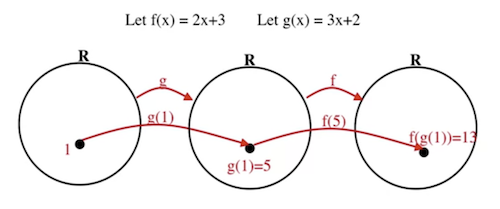• Function composition is not commutative

• $f \text{ o } g \ne g \text{ o } f$
• Example:
• $f(x) = 2x$ and $g(x) = x^2$
• $(f \text{ o } g)(x) = f(g(x)) = 2x^2$
• $(g \text{ o } f)(x) = g(f(x)) = 4x^2$
• Therefore, $f(g(x)) \ne g(f(x))$

## 2.203 Bijective function

• Bijective Function
• A function is said to be bijective or invertible if and only if it is both injective and surjective.
• Examples:
• $f$ is bijective as it is both injective and surjective.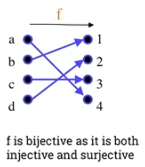• $g$ is bijective as it isn't surjective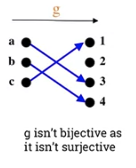• $h$ isn't bijective as it isn't injective.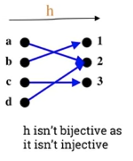• Show that the function: $f: R \rightarrow R$ with $f(x) = 2x + 3$ is a bijective (invertible) function.
• Show that f is injective (one-to-one)
• Proof:
• Let $a, b \in R$, show that if $a \ne b$ then $f(a) \ne f(b)$
• $a \ne b \Rightarrow 2a \ne 2b \Rightarrow 2a + 3 \ne 2b + 3 \Rightarrow f(a) \ne f(b)$
• Show that f is surjective (onto)
• Proof:
• Let $y \in R$ show that there exists $y \in R$ such that $f(x) = y$
• $f(x) = y \Rightarrow 2x + 3 = y \Rightarrow 2x = y - 3 \Rightarrow x = \frac{y-3}{2} \in R$
• Therefore, for every element $y$ in $R$ there exists $x$ which is $\frac{y - 3}{2}$ such that $y = f(x)$
• Since we have shown that $f(x) = 2x + 3$ is both injective and surjective, we can infer that it is a bijective function.
• Inverse Function
• Let $f: A \rightarrow B$
• If $f$ is bijective (invertible) then the inverse function, $f^{-1}$, exists and is defined as follows: $f^{-1}: B \rightarrow A$
• What the inverse function really does is reverse the function $f(x)$.
• Example 1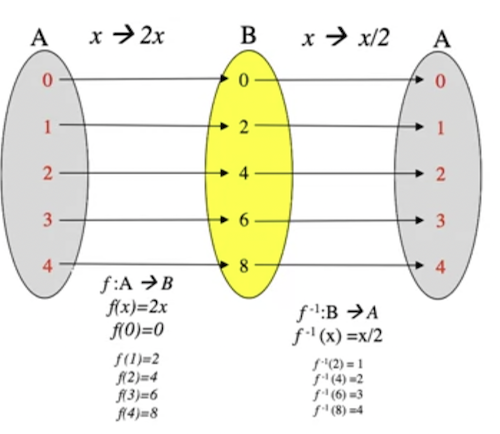• Example 2: Let $f(x) = 2x$• Exercise: The following function $f: R \rightarrow R \text{ with } f(x) = 2x + 3$ find the inverse function, $f^{-1}$
• We know it's injective and surjective.
• $f(x) = y$
• $2x +3 = y$
• $2x = y-3$
• $x = \frac{y - 3}{2}$
• $f^{-1} = R -> R$
• $x \rightarrow f^{-1} (x) = \frac{x - 3}{2}$
• A function f of its inverse is equal to the identity function:
• $(f \text{ o } f^{-1})(x) = (f^{-1} \text{ o } f)(x) = x$
• $f: R \rightarrow R \text{ with } f(x) = 2x$
• $f^1: R \rightarrow R \text{ with } f^{-1}(x) = \frac{x}{2}$
• $(f \text{ o } f^{-1})(x) = f(f^{-1}(x)) = f(\frac{x}{2}) = 2\frac{x}{2} = x$
• $(f^{-1} \text{ o } f)(x) = f^{-1}(f(x)) = f^{-1}(2x) = \frac{2x}{2} = x$
• Plotting on a graph
• The curves of $f$ and $f^{1}$ are symmetric with respect to the straight line $y = x$.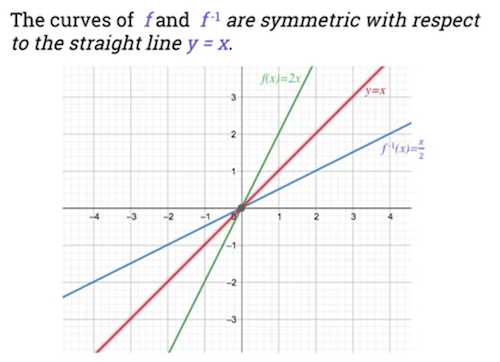## 2.205 Logarithmic functions

• Exponential Function

• $f(x) = b^x$
• The variable $b$ is called the base.
• Defined by $f : R \rightarrow R^{+}$ and $f(x) = b^{x}$ where $b > 0$ and $b \ne 1$.
• Graphs:

• First graph shows exponential growth where base > 1.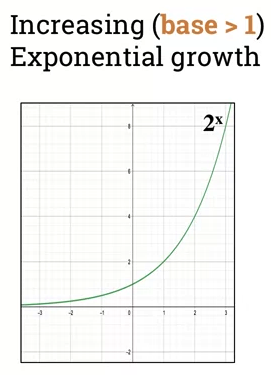• 2nd graphs exponential decay where b < 1.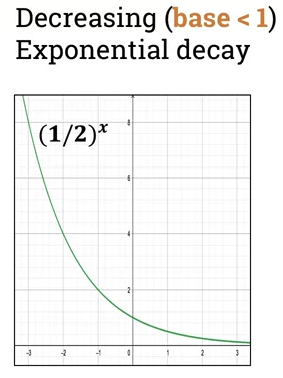• Properties of exponential function:

• $y = f(x) = b^x$ where ($b > 0$ and $b \ne 1$).
• Domain is set all real numbers: $\mathbb{R}$; $(-\infty, \infty)$
• Range is all positive real numbers: $(0, \infty)$
• The graph always passes through the point with coords $(0, 1)$
• if base is > 1, then function is increasing
• called "exponential growth"
• if base < 1, then function is decreasing
• called "exponential decay"
• it is Injective and Surjective, hence, it has an inverse function.
• Logarithmic Functions
• Logarithmic function with base b where b > 0 and $b \ne 1$ is defined as follows:
• $\log_bx = y$ if and only if $x = b^y$
• $\log_bx$ is inverse of exponential function $b^x$.
• The exponent in the exponential becomes what the log is equal to.
• Laws of Logarithms
• $\log_b m * n = log_bm + log_bn$
• $\log_b\frac{m}{n} = log_b m -log_bn$
• $\log_b m^n = n \ log_b \ m$
• $\log_b1 = 0$
• $\log_bb = 1$
• Examples
• $\log_381$
• $81 = 3^4$, so $\log_3 \ 81 = \log_3 \ 3^4$
• $\log_3 \ 3^4 = 4 \ \log_3 \ 3$
• Since $log_33$ = 1, $log_381 = 4 * 1 = 4$
• $\log_{10} \ {100} = \log_{10} \ 10^2 = 2 \ \log_{10} \ 10 = 2 * 1 = 2$
• $\log_3 \frac{1}{81} = \log_3 \frac{1}{3^4} = \log_3 \ 3^{-4} = -4 \ \log_{3}3 = -4 * 1 = -4$
• $\log_2 \ 1 = \log_2 2^0 = 0 \ log_2 \ 2 = 0 * 1 = 0$
• Examples of a logarithmic function

• $f(x) = log_2 x$
• Can see function is increasing.
• Passes through coordinate (1, 0)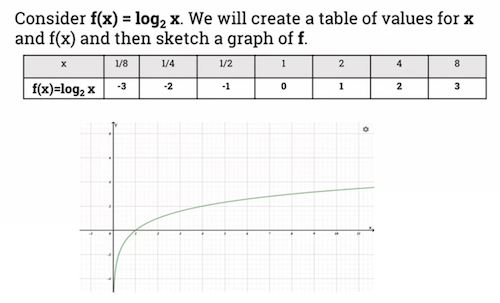• Logarithm function with b > 1

• $\log_{2} \ {x}$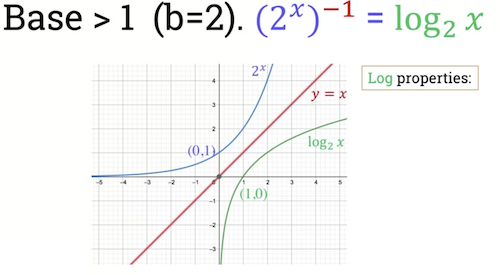• Can see the curves are symmetric with respect to red line y = x.

• This is expected as logarthim is inverse of exponential function.
• Can also infer these properties:
• Domain: $(0, \infty)$ ($\mathbb{R}^{+}$)
• Range: ($-\infty, \infty$)
• x-intercept: $(1, 0)$
• increasing on: $(0, \infty)$
• Example where b < 1

• *$log_{\frac{1}{2}} \ x$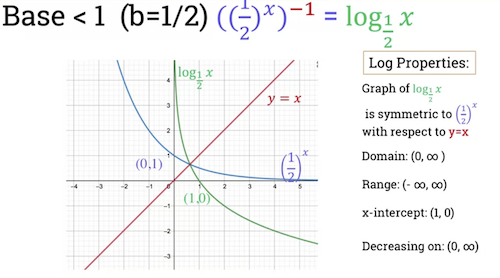• Can also infer these properties:
• Domain: $(0, \infty)$ ($\mathbb{R}^{+}$)
• Range: ($-\infty, \infty$)
• x-intercept: $(1, 0)$
• decreasing on: $(0, \infty)$
• Natural Logarithm
• Written as $\ln \ x$
• $\ln \ x = \log_e x$ where $e = 2.71828$
• $\ln e = log_e e = 1$
• Graph shows it is an increasing function:
•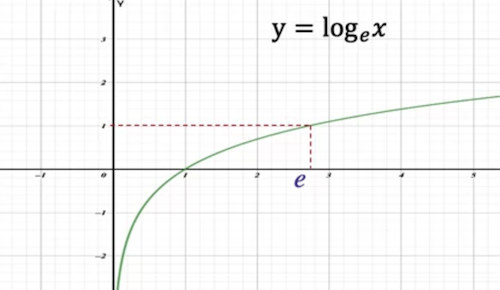## The floor function and ceiling functions

• Floor function
• Takes a real number x as input and returns the largest integer that is less than or equal to $x$
• Function domain and range: $\mathbb{R} \rightarrow \mathbb{Z}$.
• Denoted as $|\_x\_|$
• Graph of floor function:
•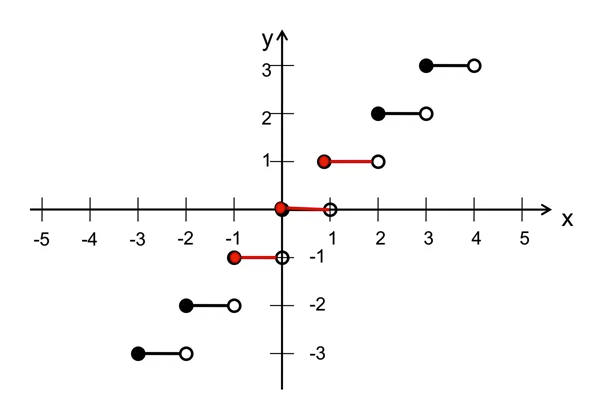• Examples:
• floor(10) = 10
• floor(1.1) = 1
• floor(1.99) = 1
• floor(-1.1) = -2
• floor(-1.99) = -2
• Ceiling function

• The opposite of the floor function.
• A function $R \rightarrow Z$
• Takes real number x as input and returns smallest integer greater than or equal to x.
• Denoted as: $\lceil{x}\rceil$
• Graph of ceiling function: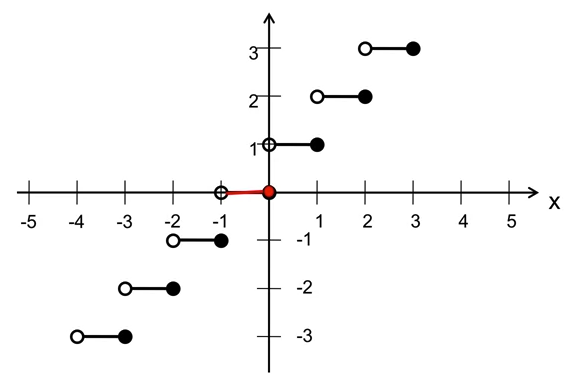• Examples:

• ceiling(10) = 10
• ceiling(1.1) = 2
• ceiling(1.99) = 2
• ceiling(-1.1) = -1
• ceiling(-1.99) = -1
• Exercise
• Let $n$ be an integer and $x$ be a real number. Show that:
• $\lfloor{x+n}\rfloor = \lfloor{x}\rfloor + n$
• Proof
• Let $m = \lfloor{x}\rfloor$
• By definition, $m \le x \lt m + 1$
• If we add inequality: $m + n \le x + n < m+n+1$
• this implies that $\lfloor{x + n}\rfloor = m + n$ (by definition)
• Hence, $\lfloor x + n \rfloor$ = $\lfloor{x}\rfloor + n$

## 2.209 Functions

Real-life examples

1. A function that is not injective (one-to-one) or surjective (onto)

A function that takes a persons name and returns their street number.

Some people live in the same house, hence not injective: $a != b$ but $f(a) == f(b)$ Also, not surjective as the co-domain is $\mathbb{R}^{+}$ but the range will not included many large numbers, depending on the size of the street.

2. A function that is injective but not surjective.

A function that takes a persons username and returns their user id, in the case where the authentication system uses an incrementing integer as the user id and the username is always unique (ala Twitter) and the range of user ids is $(0, \infty[$ A username will always map to a unique user id. However, not all user ids in the range will map to usernames, as not all possible registrations has happened yet.

3. A function that is not injective but surjective.

A function that takes all names with < 10 characters and maps to length. All images will most certainly have a preimage, however, lots of names will have the same image.

4. A function that is bijective (invertible)

A similar function to 2, however, the domain is limited to valid registered users

## Functions problem sheet

### Question 1

• Let $A$ and $B$ be two sets with $A = \{x, y, z\}$ and $B = \{1, 2, 3, 4\}$. Which of the following arrow diagrams define functions from $A$ to $B$?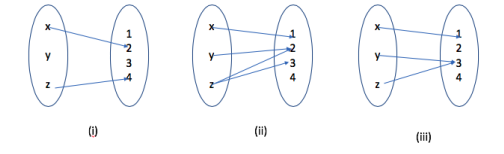• (i) is not a function as not every element in $A$ has an image in $B$.
• (ii) is not a function as some elements in A map to multiple elements in B.
• (iii) is a function, as all elements of $A$ is mapped to unique elements in $B$.

### Question 2

Let $A$ and $B$ be two sets with $A = \{x, y, z\}$ and $B = \{1, 2, 3, 4\}$.

Let $f$ from $A$ to $B$ defined by the following arrow diagram: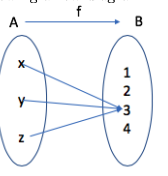1. Write the domain, the co-domain and the range of $f$.

$\text{Domain}_f = A$ $\text{Co-do}_f(x) = B$ $\text{Range}(x) = \{3\}$

2. Find $f(x)$ and $f(y)$

$f(x) = 3$ $f(y) = 3$

3. Pre-images for 3: ${x, y, z}$. Pre-images for 1: ${}$

4. $\{(x, 3), (y, 3), (z, 3)\}$

### Question 3

The Hamming Distance Function is important in coding theory.

It gives the measure of distance between 2 strings of 0's and 1's that have the same length.

Let $S_n$ be the set of all strings of 0's and 1's of length $n$.

The Humming function $H$ is defined as follows: $H: S_n \ X \ S_n => \mathbb{N} \ U \ {0}$

$(s, t) \rightarrow H(s, t)$ = the number of positions in which s and t have different values.

For n = 5, find

• $H(11111, 00000) = 5$
• $H(11000, 00000) = 2$
• $H(00101, 01110) = 3$
• $H(10001, 01111) = 4$

### Question 4

Digital messages consist of a finite sequence of 0's and 1's.

When they are communicated across a transmission channel, they are coded to reduce the chance that they will be garbled by integering noise in the transmission lines.

Let A be the set of strings of 0s and 1s and let E and D be the encoding and the decoding function on set A defined for each string. s in A as follows:

E(s) = The string obtained from s by replacing each bit of s with the same bit written three times

D(s) = The string obtained from s by replacing each consecutive triple of three identical bits of s by a single copy of that bit.

Find $E(0110)$, $E(0101)$, $D(000111000111000111111)$ and $D(111111000111000111000000)$

• *$E(0110) = 000 111 111 000$
• *$E(0101) = 000 111 000 111$
• *$D(000111000111000111111) = 0101011$
• *$D(111111000111000111000000) = 1 1 0 1 0 1 0 0$

### Question 5

Let $A = \{1, 2, 3, 4, 5, 6\}, B = \{a, b, c, d\} \text{ and } C = \{w, x, y, z\}$ be three sets.

Let $f$ and $g$ be two functions defined as follows:

$f : A \rightarrow B$ is defined by the following table:

$x$ 1 2 3 4 5 6
$f(x)$ a b a c d d

g : B → C is defined by the following table.

$x$ a b c d
$g(x)$ w x y z
1. Draw arrow diagrams to represent the function f and g.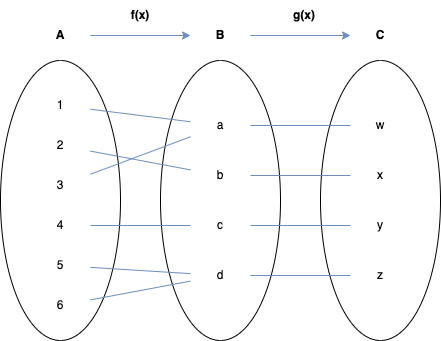2. List the domain; the co-domain and the range of f and g.

3. f(x)

• $D_f = A = \{1, 2, 3, 4, 5, 6\}$
• $\text{Co-D}_f = B = \{a, b, c, d\}$
• $R_f: \{a, b, c, d\}$\$
• set of all actual outputs, which is also co-domain.
4. $g(x)$

• $D_g = B = \{a, b, c, d\}$
• $\text{Co-D}_G = C = \{w, x, y, z\}$
• $R_g = \{w, x, y, z\}$
5. Find f(1), the ancestor (pre-image) of d. and (g o f)(3)

6. $f(1) = a$

7. $d = {5, 6}$
8. $g(f(3)) = w$

9. Show that f is not a one to one function.

10. $f(x)$ is not injective as $f(1) = f(3)$.

11. Show that f is an onto function.

12. $f(x)$ is surjective as all $x \in B$ have at least one pre-image.

13. Show that g is both one to one and onto

14. $g(x)$ is injective as $\forall x \in C$, if $x \ne y$ then $g(x) \ne g(y)$

15. $g(x)$ is surjective as all $x \in C$ has at a pre-image.

### Question 6

Suppose you read that a function $f : \mathbb{Z} \times \mathbb{Z}^{+} \rightarrow \mathbb{Q}$ is defined by the formula $f(m, n) = \frac{m}{n}$ for all $(m, n) \in \mathbb{Z} \times \mathbb{Z}^{+}$

• $f$ is a not a one-to-one function. f(1, 1) = f(2, 2).
• Every ratioal number can be writen with a positive denominator, hence, f is an onto function.

### Question 7

Given a function $f$ defined by $f(x) = \lfloor x \rfloor$ where $f : \mathbb{R} \rightarrow \mathbb{Z}$

1. Plot the graph of a the function $f(x)$ where $x \in [-3, 3]$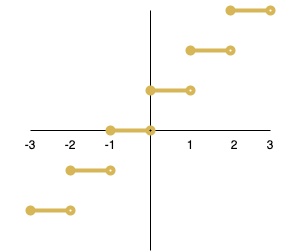2. Find $floor(\pi)$, $floor(-2.5)$, $floor(-1)$

3. $\lfloor \pi \rfloor = 3$

4. $\lfloor -2.5 \rfloor = -3$
5. $\lfloor -1 \rfloor = -1$

6. Show that $f$ is not injective

We can see that $f(1.5) = f(1.6) = 1$, therefore, the function is not injective.

1. Show that f is surjective.

For a $n \in \mathbb{Z}$ there exists at least one pre-image $x$ in $\mathbb{R}$ such that $\lfloor x \rfloor = n$.

Therefore every element of the co-domain has a pre-image, and the floor function is an onto function.